# NCERT Solutions for Class 12 Maths Chapter 10 Vector Algebra Miscellaneous Exercise

These NCERT Solutions for Class 12 Maths Chapter 10 Vector Miscellaneous Exercise Questions and Answers are prepared by our highly skilled subject experts.

## NCERT Solutions for Class 12 Maths Chapter 10 Vector Miscellaneous ExerciseQuestion 1.
Write down a unit vector in XY – plane, making an angle of 30° with the positive direction of x – axis.
Solution:
Let P(x, y) be any point on the line making 30° with x-axis. Let $$\vec{a}$$ = x$$\hat{i}$$ + y$$\hat{i}$$ be a unit vector.Another method:
The line in the above figure lies in X Y plane and makes 30° with positive direction of x – axis. Hence the line makes 60° with y- axis and 90° with z – axis.
The direction cosines of the line
= cos 30°, cos 60°, cos 90° = $$\frac{\sqrt{3}}{2}$$, $$\frac { 1 }{ 2 }$$, 0
Hence the unit vector is $$\frac{\sqrt{3}}{2}$$$$\hat{i}$$ + $$\frac { 1 }{ 2 }$$$$\hat{j}$$ + 0$$\hat{k}$$.

Question 2.
Find the scalar components and magnitude of the vector joining the points P(x1, y1, z1) and Q(x2, y2, z2).
Solution: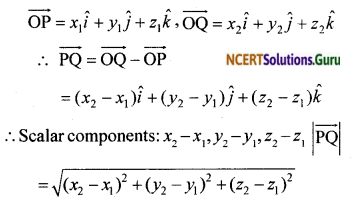Question 3.
A girl walks 4 km towards west, then she walks 3 km in a direction 30° east of north and stops. Determine the girl’s displacement from her initial point of departure.
Solution: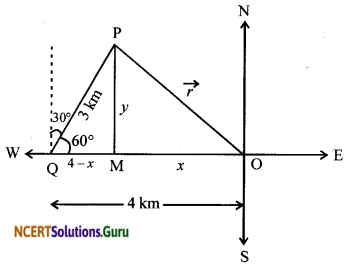Let the girl starts from O and stops at P. Let the coordinates of P be (x, y).
$$\vec{r}$$ = $$\overrightarrow{OP}$$
∴ $$\vec{r}$$ = – x$$\hat{i}$$ + y $$\hat{j}$$ … (1)
In ∆ QMP, sin60° = $$\frac { y }{ 3 }$$Question 4.
If $$\vec{a}$$ = $$\vec{b}$$ + $$\vec{c}$$, then is it true that |$$\vec{a}$$| = |$$\vec{b}$$| + |$$\vec{c}$$|? Justify your answer.
Solution:Question 5.
Find the value of x for which x$$(\hat{i}+\hat{j}+\hat{k})$$ is a unit vector.
Solution:Question 6.
Find a vector of magnitude 5 units, and parallel to the resultant of the vectors $$\vec{a}=2 \hat{i}+3 \hat{j}-\hat{k} \text { and } \vec{b}=\hat{i}-2 \hat{j}+\hat{k}$$.
Solution:
$$\vec{a}+\vec{b}$$ be the resultant of $$\vec{a}$$ and $$\vec{b}$$.Question 7.
If $$\vec{a}$$ = $$\hat{i}+\hat{j}+\hat{k}$$, $$\vec{b}$$ = $$2 \hat{i}-\hat{j}+3 \hat{k}$$ and $$\vec{c}$$ = $$\hat{i}-2 \hat{j}+\hat{k}$$, find a unit vector parallel to the vector $$2 \vec{a}-\vec{b}+3 \vec{c}$$.
Solution: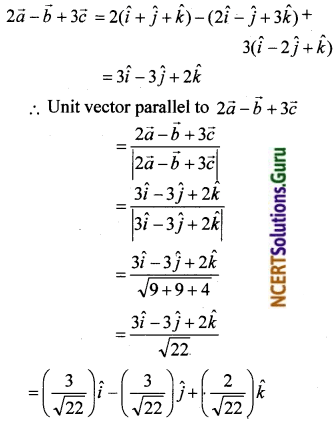Question 8.
Show that the points A (1, – 2, – 8), B (5, 0, – 2)and C (11, 3, 7) are collinear and find the ratio in which B divides AC.
Solution: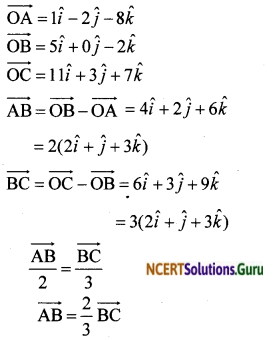$$\overrightarrow{AB}$$ is a scalar multiple of $$\overrightarrow{BC}$$
∴ $$\overrightarrow{AB}$$ || $$\overrightarrow{BC}$$ and B is the common point.
Hence A, B, C are collinear.
Also $$\frac{|\overrightarrow{\mathrm{AB}}|}{|\overrightarrow{\mathrm{BC}}|}=\frac{2}{3}$$
∴ The point B divides AC in the ratio 2 : 3.

Another method:
Let $$\vec{a}$$, $$\vec{b}$$ and $$\vec{c}$$ be the position vectors of A, B and C.
∴ $$\vec{a}$$ = $$\hat{i}-2 \hat{j}-8 \hat{k}$$, $$\vec{b}$$ = $$5 \hat{i}+0 \hat{j}-2 \hat{k}$$, $$\vec{c}$$ = $$11 \hat{i}+3 \hat{j}+7 \hat{k}$$
Let B divide AC in the ratio λ : 1
By section formula, the position vector ofEquating components of $$\hat{i}$$, $$\hat{j}$$ and $$\hat{k}$$, we get∴ λ = $$\frac{2}{3}$$ satisfies (1) and (3)
Hence A, B and C are collinear and B divides AC in the ratio $$\frac{2}{3}$$ : 1 That is, in the ratio 2 : 3.Question 9.
Find the position vector of a point R which divides the line joining two points P and Q whose position vectors are (2$$\vec{a}$$ + $$\vec{b}$$) and ($$\vec{a}$$-3$$\vec{b}$$) externally in the ratio 1 : 2. Also, show that P is the midpoint of the line, segment RQ.
Solution:
$$\overrightarrow{\mathrm{OP}}=2 \vec{a}+\vec{b}, \overrightarrow{\mathrm{OQ}}=\vec{a}-3 \vec{b}$$,
R divides PQ in the ratio 1 : 2 externally.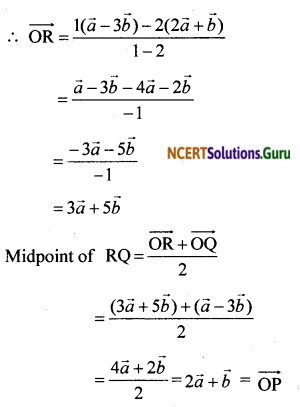∴ P is the midpoint of the line segment RQ.

Question 10.
The two adjacent sides of a parallelogram are $$2 \hat{i}-4 \hat{j}+5 \hat{k} \text { and } \hat{i}-2 \hat{j}-3 \hat{k}$$. Find the unit vector parallel to its diagonal. Also, find its area.
Solution:
Let $$\vec{a}=2 \hat{i}-4 \hat{j}+5 \hat{k} \text { and } \vec{b}=\hat{i}-2 \hat{j}-3 \hat{k}$$ be any two adjacent sides of a parallelogram.
$$\vec{a}$$ + $$\vec{b}$$ is a vector along the diagonal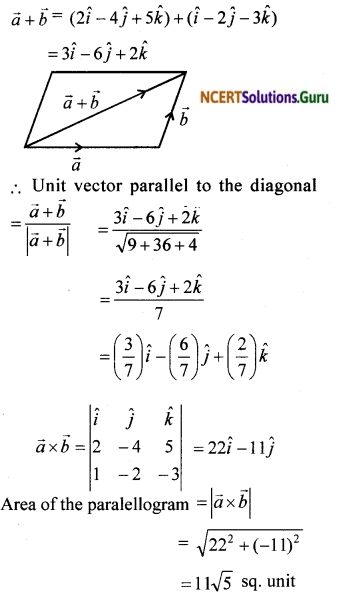Question 11.
Show that the direction cosines of a vector equally inclined to the axes OX, OY and OZ are $$\frac{1}{\sqrt{3}}, \frac{1}{\sqrt{3}}, \frac{1}{\sqrt{3}}$$.
Solution:
Let a, P and y be the angles made by the vector with the axes OX, OY and OZ.
Let l, m and n be the direction cosines.
Since the vector is equally inclined to the
axes, α = ß = γ
∴ l = cosα, m = cos α, n = cos α
We have l² + m² + n² = 1
i.e, cos² α + cos² α + cos² α = 1
⇒ 3 cos² α = 1
cos² α = $$\frac { 1 }{ 3 }$$
cos α = $$\frac{1}{\sqrt{3}}$$
∴ l = m = n = $$\frac{1}{\sqrt{3}}$$
Hence the direction cosines of the vector equally inclined to the axes are $$\frac{1}{\sqrt{3}}$$, $$\frac{1}{\sqrt{3}}$$, $$\frac{1}{\sqrt{3}}$$.Question 12.
Let $$\vec{a}=\hat{i}+4 \hat{j}+2 \hat{k}, \vec{b}=3 \hat{i}-2 \hat{j}+7 \hat{k}$$ and $$\vec{c}=2 \hat{i}-\hat{j}+4 \hat{k}$$ Find a vector $$\vec{d}$$ which is perpendicular to both $$\vec{a}$$ and $$\vec{b}$$ and $$\vec{c}$$. $$\vec{d}$$ = 15.
Solution:
Let $$\vec{d}$$ = $$x \hat{i}+y \hat{j}+z \hat{k}$$
Since $$\vec{d}$$ is perpendicular to both $$\vec{a}$$ and $$\vec{b}$$
$$\vec{d}$$.$$\vec{a}$$ = 0 and $$\vec{d}$$.$$\vec{b}$$ = 0.
x + 4y + 2z = 0 … (1) and
3x – 2y + 7z = 0 … (2)
Given $$\vec{c}$$.$$\vec{d}$$ = 15 … (3)
2x – y + 4z = 15 … (3)
Solving (1), (2) and (3), we get x = $$\frac { 160 }{ 3 }$$
y = $$\frac { – 5 }{ 3 }$$ and z = $$\frac { -70 }{ 3 }$$
∴ $$\vec{d}=\frac{160}{3} \hat{i}-\frac{5}{3} \hat{j}-\frac{70}{3} \hat{k}$$
$$\vec{d}=\frac{1}{3}(160 \hat{i}-5 \hat{j}-70 \hat{k})$$

Question 13.
The scalar product of the vector $$\hat{i}+\hat{j}+\hat{k}$$ with a unit vector along the sum of vectors $$2 \hat{i}+4 \hat{j}-5 \hat{k} \text { and } \lambda \hat{i}+2 \hat{j}+3 \hat{k}$$ is equal to one. Find the value of λ.
Solution: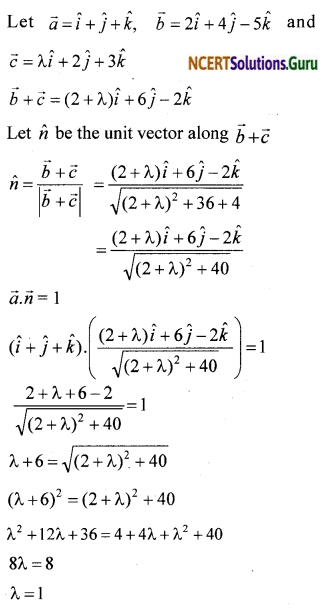Question 14.
If $$\vec{a}$$, $$\vec{b}$$ and $$\vec{c}$$ are mutually perpendicular vectors of equal magnitudes, show that the vectors $$\vec{a}+\vec{b}+\vec{c}$$ is equally inclined to $$\vec{a}$$, $$\vec{b}$$ and $$\vec{c}$$.
Solution:
Given that $$|\vec{a}|=|\vec{b}|=|\vec{c}|=\lambda$$ … (1)
Since $$\vec{a}$$, $$\vec{b}$$ and $$\vec{c}$$ are mutually perpendicular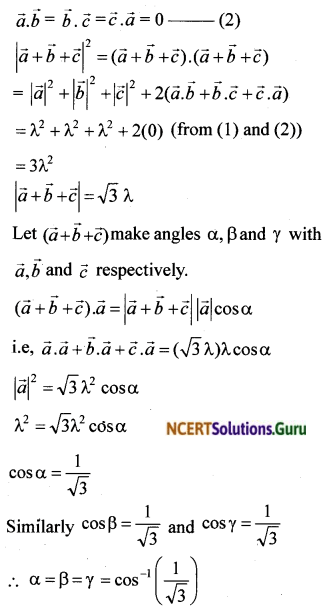Hence $$\vec{a}+\vec{b}+\vec{c}$$ is equally inclined to $$\vec{a}$$, $$\vec{b}$$ and $$\vec{c}$$.Question 15.
Prove that $$(\vec{a}+\vec{b}) \cdot(\vec{a}+\vec{b})=|\vec{a}|^{2}+|\vec{b}|^{2}$$, if and only if $$\vec{a}$$, $$\vec{b}$$ are perpendicular, given a ≠ $$\vec{0}$$, b ≠ $$\vec{0}$$
Solution:
$$(\vec{a}+\vec{b}) \cdot(\vec{a}+\vec{b})=\vec{a} \cdot \vec{a}+\vec{a} \cdot \vec{b}+\vec{b} \cdot \vec{a}+\vec{b} \cdot \vec{b}$$
$$(\vec{a}+\vec{b}) \cdot(\vec{a}+\vec{b})=|\vec{a}|^{2}+|\vec{b}|^{2}+2(\vec{a} \cdot \vec{b}) \ldots \ldots$$ … (1)
Case 1
Let
From (1), we get $$\vec{a}$$, $$\vec{b}$$ = 0
Hence $$\vec{a}$$ ⊥ $$\vec{b}$$ since $$\vec{a}$$ and $$\vec{b}$$ are nonzero vectors.

Case 2
Let $$\vec{a}$$ ⊥ $$\vec{b}$$. Then $$\vec{a}$$.$$\vec{b}$$ = 0
(1) → $$(\vec{a}+\vec{b}) \cdot(\vec{a}+\vec{b})=|\vec{a}|^{2}+|\vec{b}|^{2}$$ + 0
$$(\vec{a}+\vec{b}) \cdot(\vec{a}+\vec{b})=|\vec{a}|^{2}+|\vec{b}|^{2}$$

Question 16.
If θ is the angle between two vectors $$\vec{a}$$ and $$\vec{b}$$, then $$\vec{a}$$.$$\vec{b}$$ ≥ 0 only when
a. 0 < θ < $$\frac { π }{ 2 }$$
b. 0 ≤ θ ≤ $$\frac { π }{ 2 }$$
c. 0 < θ < π
d. 0 ≤ θ ≤ π
Solution: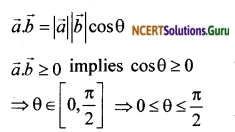Question 17.
Let $$\vec{a}$$ and $$\vec{b}$$ be two unit vectors and θ is the angle between them. Then $$\vec{a}$$ + $$\vec{b}$$ is a unit vector if
a. θ = $$\frac { π }{ 4 }$$
b. θ = $$\frac { π }{ 3 }$$
c. θ = $$\frac { π }{ 2 }$$
d. θ = $$\frac { 2π }{ 3 }$$
Solution:Question 18.
The value of
$$\hat{i} \cdot(\hat{j} \times \hat{k})+\hat{j} \cdot(\hat{i} \times \hat{k})+\hat{k} \cdot(\hat{i} \times \hat{j}) \text { is }$$
a. 0
b. -1
c. 1
d. 3
Solution:
c. 1
$$\hat{i} \cdot(\hat{j} \times \hat{k})+\hat{j} \cdot(\hat{i} \times \hat{k})+\hat{k} \cdot(\hat{i} \times \hat{j}) \text { is }$$
= $$\hat{i} \cdot \hat{i}-\hat{j} \cdot \hat{j}+\hat{k} \hat{k}$$
= 1 – 1 + 1 = 1Question 19.
If θ is the angle between any two vectors $$\vec{a}$$ and $$\vec{b}$$, then $$|\vec{a} \cdot \vec{b}|=|\vec{a} \times \vec{b}|$$, when θ is equal to
a. 0
b. $$\frac { π }{ 4 }$$
c. $$\frac { π }{ 2 }$$
d. π
Solution:
b. $$\frac { π }{ 4 }$$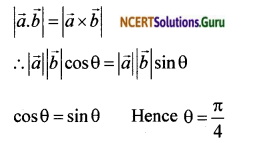error: Content is protected !!